# Test Prep Plan - Take a practice test

Take this practice test to check your existing knowledge of the course material. We'll review your answers and create a Test Prep Plan for you based on your results.
How Test Prep Plans work
1
2Based on your results, we'll create a customized Test Prep Plan just for you!
3Study smarter
Study more effectively: skip concepts you already know and focus on what you still need to learn.

# FTCE Math: Calculating Derivatives & Derivative Rules Chapter Exam

Exam Instructions:

Choose your answers to the questions and click 'Next' to see the next set of questions. You can skip questions if you would like and come back to them later with the yellow "Go To First Skipped Question" button. When you have completed the practice exam, a green submit button will appear. Click it to see your results. Good luck!

### Page 1

#### Question 1 1. Find the jerk of the function shown below: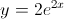#### Question 2 2. Find the derivative of p(t).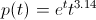#### Question 3 3. What is the highest-order derivative of f(x) that is NOT zero?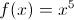#### Question 4 4. The graph x(t) represents the position of an object as a function of time. What is the acceleration of the object for t>0?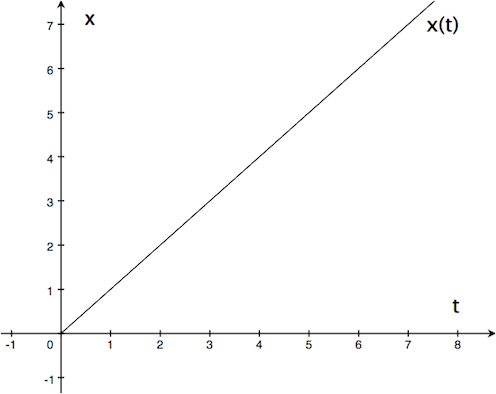#### Question 5 5. Evaluate the following.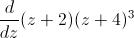### Page 2

#### Question 6 6. If the following graph is f(x), then which of the graphs below could be f''(x)?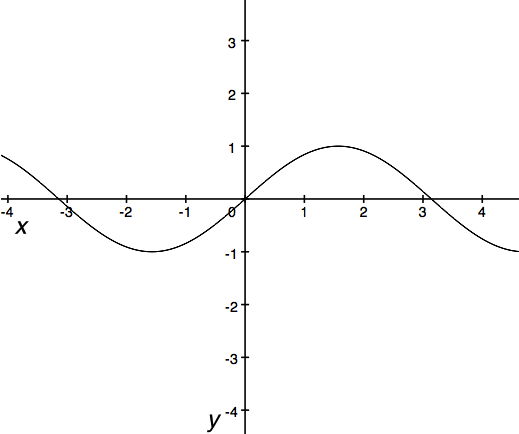#### Question 7 7. If h equals 3 m, and x is moving at a rate of 3 m / second, how fast is L changing when x equals 4m?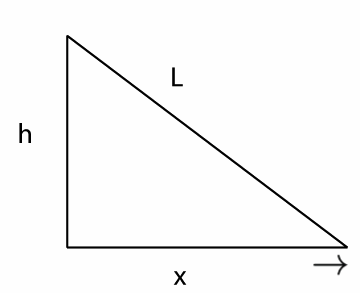#### Question 8 8. Find the derivative df/dk.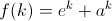#### Question 9 9. Find the derivative of f(x).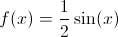#### Question 10 10. Find the derivative of f(x).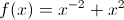### Page 3

#### Question 11 11. The red and blue cars are 10 miles apart, but headed toward one another. The red car is traveling at 45 mph, and the blue car is traveling at a speed v(t). What is the equation for how the distance between them changes as a function of time?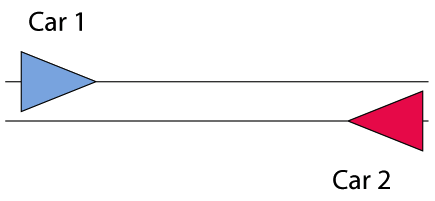#### Question 12 12. What is the derivative of f(x)?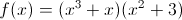#### Question 13 13. Find the second derivative of y(x).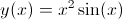#### Question 14 14. Find the derivative of f(x).#### Question 15 15. What is the equation of how L changes as a function of how x changes if h=6?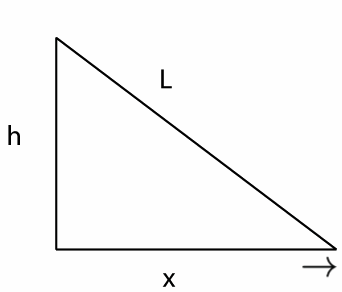### Page 4

#### Question 17 17. Evaluate.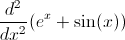#### Question 18 18. What is the derivative of f(x)?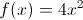#### Question 19 19. Calculate the derivative of g(t).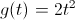#### Question 20 20. Find the derivative of f(x).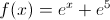### Page 5

#### Question 21 21. What is the derivative of f(x)?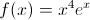#### Question 22 22. If h(t) is the height of an object as a function of time, then which of the following statements are true about h(t)? (Assume t>0.) A) When hitting the ground (at h(t)=0), the object's velocity is -2. B) When hitting the ground (at h(t)=0), the object's acceleration is -2. C) The object hits the ground at t=2.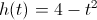#### Question 23 23. Find the derivative of f(x).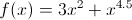#### Question 24 24. Evaluate.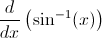#### Question 25 25. Find f'(x) for the following function.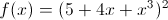### Page 6

#### Question 26 26. Given the derivative of a function (ƒ′(x)), which of the following could be ƒ(x)?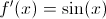#### Question 27 27. Evaluate the following.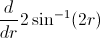#### Question 28 28. Find the derivative of f(t).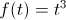#### Question 29 29. Find the derivative df/dt.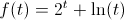#### Question 30 30. What is the derivative of f(t)?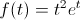#### FTCE Math: Calculating Derivatives & Derivative Rules Chapter Exam Instructions

Choose your answers to the questions and click 'Next' to see the next set of questions. You can skip questions if you would like and come back to them later with the yellow "Go To First Skipped Question" button. When you have completed the practice exam, a green submit button will appear. Click it to see your results. Good luck!

Support